## “Understanding bias and variance trade off, which have roots in mathematics and statistics, is essential for training Artificial Neural Networks. Bias and variance are used in supervised learning problems in which an algorithm learns from training data or a sample data set of known quantities. The correct balance of bias and variance is vital to building Artificial Neural Networks of different sizes, that create accurate results from such models.”

It is well known that we can train Neural Networks of different layers using datasets of different sizes. But is it necessary to get as good a result out of your Neural Network model as you wish? Can I get an ideal 100% result out of my model? The answer is no for most of the time. We know that Neural Networks behave similar to a function which maps the input data to its corresponding output. As the output provided by a Neural Network is an approximation to the actual output, there is always an error term involved in such approximations. The basic idea is that we have training data to train the Neural Network and we test the performance of our trained network on the testing data as a consequence of which we have different errors on training and testing data, these errors have a direct relation to the bias and variance of the model and we can use it as a measure to judge the balance of our model.

What is Bias?

The bias is known as the difference between the prediction of the values by the Neural Network model and the correct value. A high bias model gives a large error in training as well as testing data. It is recommended that an algorithm should always be low biased to avoid the problem of underfitting. Also called “error due to squared bias”, bias is the amount that a model’s prediction differs from the target value, compared to the training data. Bias error results from simplifying the assumptions used in a model so the target functions are easier to approximate. Bias can be introduced by model selection. Every algorithm starts with some level of bias, because bias results from assumptions in the model that make the target function easier to learn. A high level of bias can lead to underfitting, which occurs when the algorithm is unable to capture relevant relations between features and target outputs. A high bias model typically includes more assumptions about the target function or end result. A low bias model incorporates fewer assumptions about the target function.

A linear algorithm often has high bias, which makes them learn fast. In linear regression analysis, bias refers to the error that is introduced by approximating a real-life problem, which may be complicated, by a much simpler model. Though the linear algorithm can introduce bias, it also makes their output easier to understand. The simpler the algorithm, the more bias it has likely introduced. In contrast, nonlinear algorithms often have low bias.

As an example to visualize a bias in the model, refer to the figure below: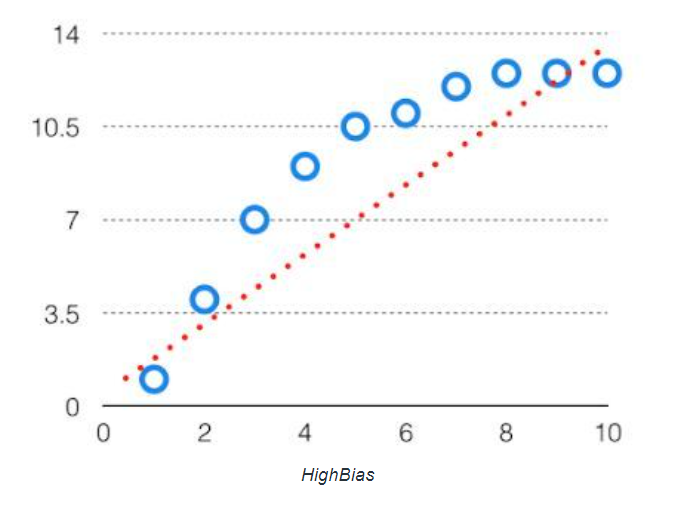By high bias, the data predicted is in a straight line format, thus not fitting accurately in the data in the data set. This type of fitting is called the Underfitting of Data. This happens when the hypothesis is too simple or linear in nature.

In this case the hypothesis looks like: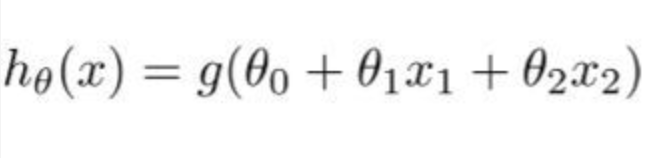What is Variance?

The Variance of a model is the variability of model prediction for a given data point which tells us about spread of our data. The model with high variance has a very complex fit to the training data and thus is not able to fit accurately on the data which it hasn’t seen before; ie the test data. As a result, such models perform very well on training data but have high error rates on test data.

When a model is high on variance, then the model is said to be Overfitting on the Data. Overfitting is fitting the training set accurately via complex curve and high order hypothesis but is not the solution as the error with unseen data is high. Variance can lead to Overfitting, in which small fluctuations in the training set are magnified. A model with high-level variance may reflect random noise in the training data set instead of the target function. The model should be able to identify the underlying connections between the input data and variables of the output. While training on data, we always aim that the model should not overfit on it. A model with low variance means sampled data is close to where the model predicted it would be. A model with high variance will result in significant changes to the projections of the target function.

An example of high variance data can be shown as below: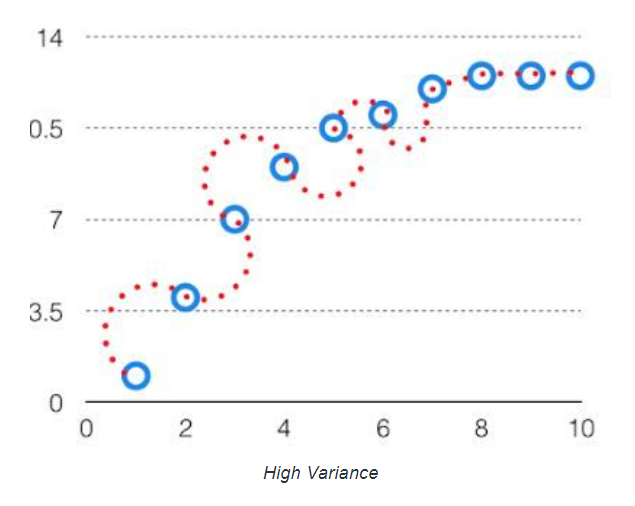In this case, the hypothesis contains higher degree polynomial terms and hence makes the curve more complex: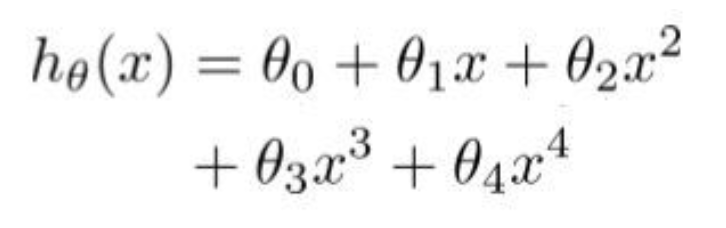Bias vs Variance Tradeoff while training Neural Networks:

It refers to the fact that when we try to make a statistical prediction using a neural network, we find a tradeoff between its bias and variance. But, one may be curious as to how we observe a tradeoff between these? Lets understand it using some intuitive example: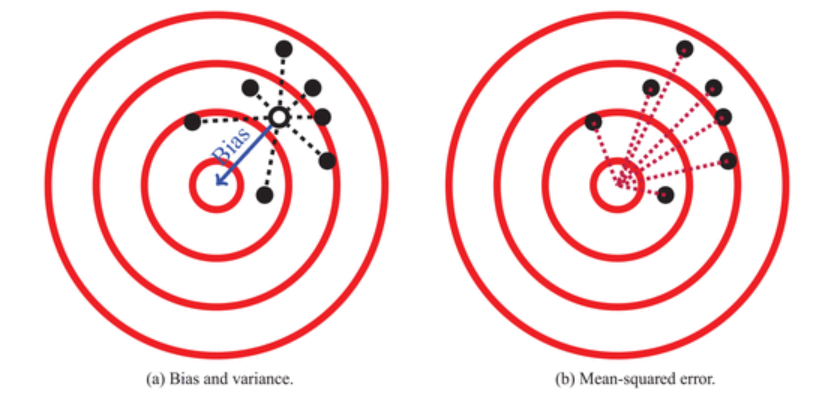Take an example of a process which generates points on a target, a good example of it would be an archer shooting arrows at the target as shown by the figure above. The shots are represented by black dots and the archer wants to hit as close to the center as possible. The bias of the process is how far the points are from the center of the target on average, as shown by the blue solid line, and the variance of the process is a measure of how far the points are to the centroid of the points on average, as depicted by the black dotted lines. Precisely, the variance can be estimated by taking the mean of the squared distances from each point to the centroid of the points (Shown by dotted lines in the left figure). Now suppose an archer has only one shot at the target and wants to be as close to the center as possible. One way to measure how far the archer’s shot will likely be from the center is the mean squared error, which is the average distance from a point to the center of the target, which can be estimated as the mean of the squared distances between all the points and the center of the target (Shown in the right figure). The mean squared error increases as the bias or variance increases. We can decompose the mean squared error into bias and variance terms as shown below: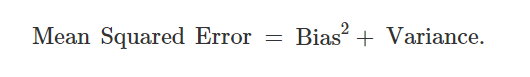The aim is to estimate a function (by training a neural network on our data) that minimizes the mean squared error distance between the estimated function and the true function.

The figures above show examples of low bias and low variance, high bias and low variance, low bias and high variance, and both high bias and high variance.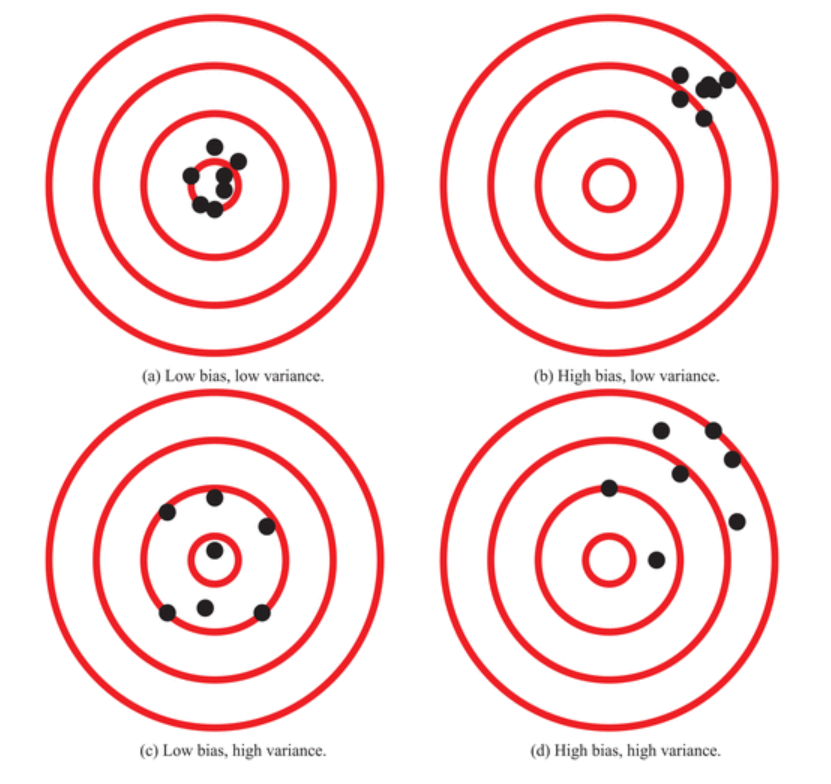We try to train a Neural Network in a way that the estimated function balances the bias-variance tradeoff, the one which captures the target function well. However, as we do not know the form of the target function beforehand, it is not always possible. Suppose we are trying to predict some target function f : RmR, suppose y = f(x), where x = (x1,x2,…xm). We use dataset D = {(xi,yi)} PD to fit an estimator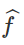of the target f by training a neural network model. For any x ∈ Rm, the bias of the predictor’s function class is the difference between the expected value of the predictor and the expected value of the target at x.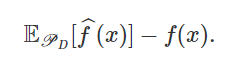For a given x, the variance of the predictor’s function class is the expected difference between the value of the predictor estimated on a randomly sampled data set and the expected value of the predictor shown as below:Mean squared error will be represented as: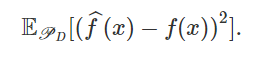As we can decompose the Mean squared error into bias and variance, it can be represented as :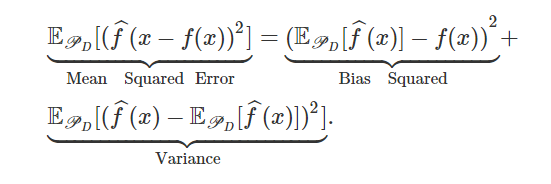This decomposition gives us a sense of why we experience bias-variance tradeoff in neural networks. For two function classes that have the same prediction error, if one function class is more biased than the other, then we know the other must have higher variance. Naturally, many neural network models tend to be more susceptible to either bias or variance.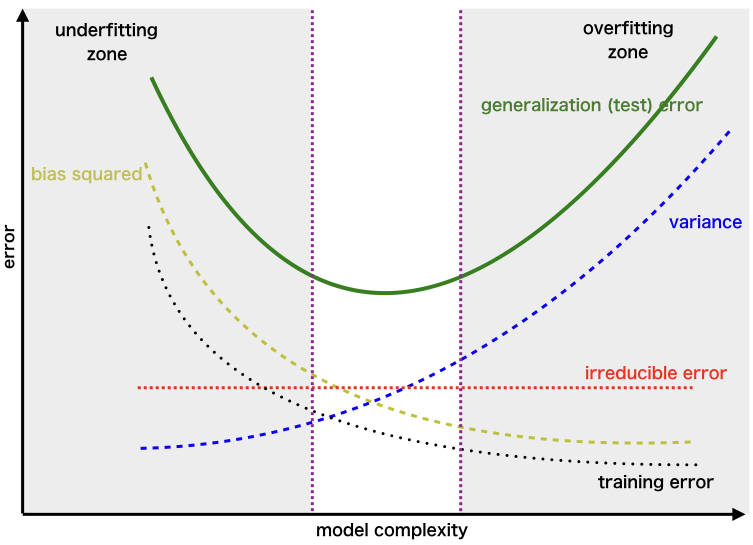The above figure shows how bias, variance, and mean squared error tend to change as a function of model complexity. Increasing model complexity could correspond to increasing the number of features in a linear regression, increasing the highest degree in a polynomial regression, or increasing the number of layers in a neural network. As the complexity increases, the bias tends to decrease but the variance tends to increase. There is typically a degree of complexity where the mean squared error is minimized by effectively balancing bias and variance. We want our model complexity to lie in the narrow region shown in between two vertical dotted lines to perform good both on our train as well as test dataset. The irreducible error is the one which is inherent and cannot be removed by modeling.

How to reduce underfitting present in a Neural Network?

• Increase the complexity of the model: The model may be underfitting simply because it is not complex enough to capture patterns in the data. Using a more complex model, for instance by switching from a linear to a non-linear model or by adding hidden layers to your neural network, will very often help solve underfitting. Increasing the complexity of the model hence helps to better capture the patterns in the data.

Possible ways to increase the complexity in a Neural Network are:

→ Increase the number of layers in the model.

→ Increase the number of neurons in each layer.

→ Change what type of layers we are using and its location. (We may also try to change the activations present in between the layers and check which one is able to “fire” better.)

• Adding more features to the Input Sample (Training Data): In contrast to overfitting, the model may be underfitting because the training data may be too simple. It may lack the features that will make the model detect the relevant patterns to make accurate predictions. Adding features and complexity to your data can help overcome underfitting.

For example, say we have a model that is attempting to predict the price of a stock based on the last three closing prices of this stock. So our input would consist of three features:

→ Day 1 Price

→ Day 2 Price

→ Day 3 Price

If we add features to this data like opening price of the day, the volume of stock for these days etc, then these added features help the model to learn more about the data and improve its accuracy.

How to reduce overfitting present in a Neural Network?

• Simplifying the Model: The first step when dealing with overfitting is to decrease the complexity of the model. To decrease the complexity, we can simply remove layers or reduce the number of neurons to make the network smaller. While doing this, it is important to calculate the input and output dimensions of the various layers involved in the neural network. There is no general rule on how much to remove or how large your network should be. But, if your neural network is overfitting, try making it smaller.

• Use Regularization: Regularization is a technique to reduce the complexity of the model. It does so by adding a penalty term to the loss function. Due to the addition of this penalty term, the values of weight matrices decrease because it assumes that a neural network with smaller weight matrices leads to simpler models. Therefore, it will also reduce overfitting to quite an extent. The most common techniques are known as L1 and L2 regularization:

L1 Regularization: The L1 penalty aims to minimize the absolute value of the weights. The mathematical formula for loss function can be represented as below. It produces a model that is simple and interpretable and is robust to outliers.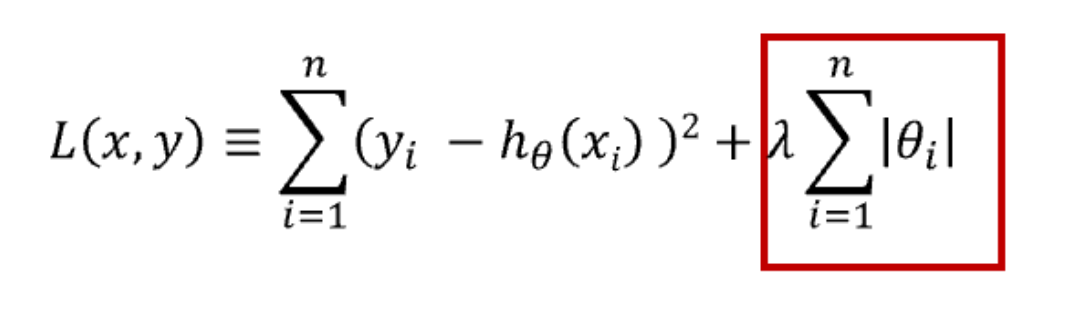→ L2 Regularization: The L2 penalty aims to minimize the squared magnitude of the weights. The mathematical formula for loss function can be represented as below. It produces a model that is able to learn complex data patterns but may not be robust to outliers.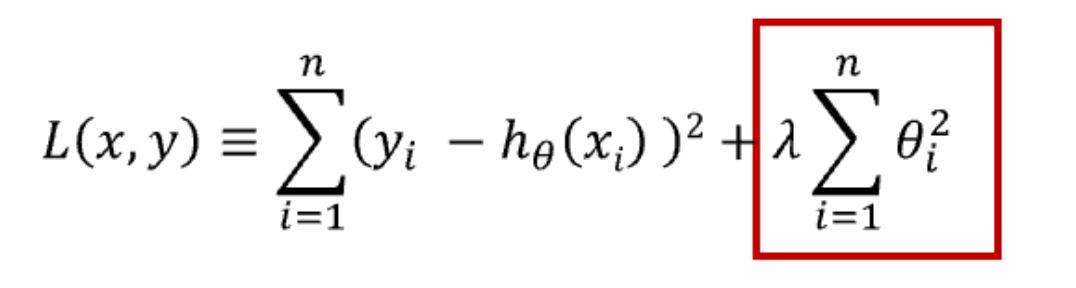Which type of regularization should one choose over the other? – If the data is too complex to be modeled accurately then L2 is a better choice as it is able to learn inherent patterns present in the data. While L1 is better if the data is simple enough to be modeled accurately. For most of the computer vision problems, L2 regularization almost always gives better results. However, L1 has an added advantage of being robust to outliers. So the correct choice of regularization depends on the problem that we are trying to solve.

• Early Stopping: It is a form of regularization while training a model with an iterative method, such as gradient descent. Since all the neural networks learn exclusively by using gradient descent, early stopping is a technique applicable to all the problems. This method updates the model so as to make it better fit the training data with each iteration. Up to a point, this improves the model’s performance on data on the test set. Past that point however, improving the model’s fit to the training data leads to increased generalization error. Early stopping rules provide guidance as to how many iterations can be run before the model begins to overfit.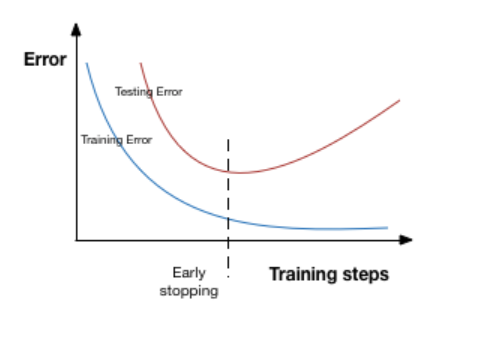In the above image, we will stop training at the dotted line since after that our model will start overfitting on the training data.

• Using Dropouts: Dropout is a regularization technique that prevents neural networks from overfitting. Regularization methods like L1 and L2 reduce overfitting by modifying the cost function. Dropout on the other hand, modify the network itself. It randomly drops neurons from the neural network during training in each iteration. When we drop different sets of neurons, it’s equivalent to training different neural networks. The different networks will overfit in different ways, so the net effect of dropout will be to reduce overfitting.

Network without Dropout: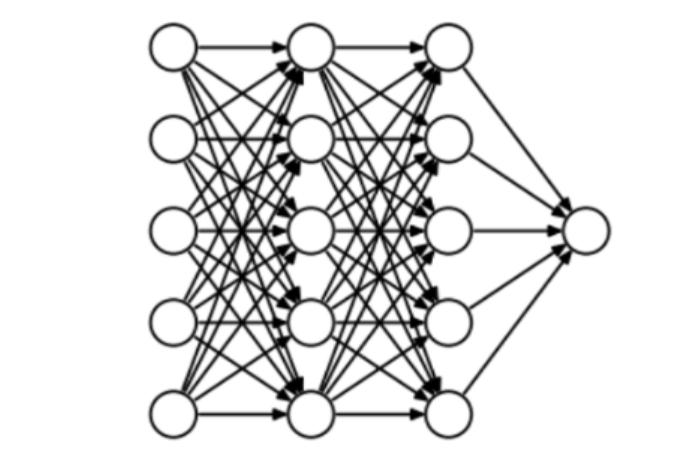Network with Dropout: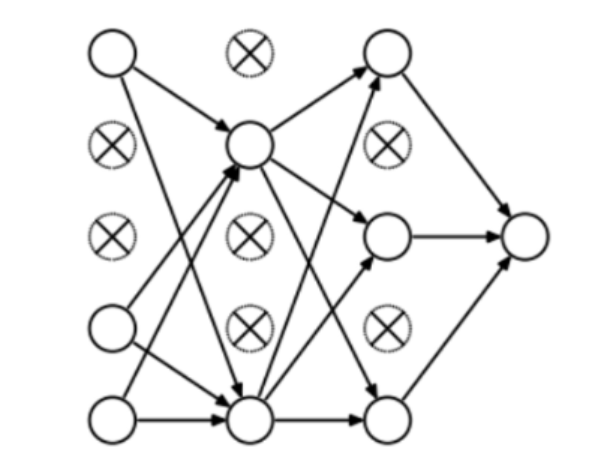At every iteration, it randomly selects some nodes with a probability(p) of choosing a particular node and removes them along with all of their incoming and outgoing connections as shown below.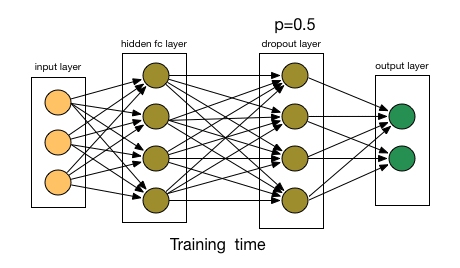Example Code for using Dropout in Neural Networks:

				
@file:DependsOn("org.jetbrains.kotlinx:kotlin-deeplearning-api:0.2.0")//Deeplearning api
//Important keyword to use s2
%use s2


				
// Some important import statements required for the code
import java.util.* // Accessing all classes and methods in Java
import kotlin.* // Accessing all classes and methods in Kotlin
import kotlin.jvm.JvmStatic // Specifies that an additional static method needs to be generated from this element if this is
// a function

/*Important modules required to be included from Kotlinx of Maven Repository
for creating and customizing your own neural network and then training it.*/

import org.jetbrains.kotlinx.dl.dataset.* // Accessing all the pre-available datasets
import org.jetbrains.kotlinx.dl.api.core.Sequential // Accessing the sequential layers for creating neural network models
import org.jetbrains.kotlinx.dl.api.core.layer.regularization.Dropout // Accessing the Dropout layer for regularizing the Neural Network
import org.jetbrains.kotlinx.dl.api.core.layer.core.Dense // Accessing the dense layers
import org.jetbrains.kotlinx.dl.api.core.layer.core.Input // Accessing the input layers
import org.jetbrains.kotlinx.dl.api.core.layer.reshaping.Flatten // Accessing the flatten layers for converting a 2-D image to 1-D
// layer of units
import org.jetbrains.kotlinx.dl.api.core.loss.Losses // Accessing the loss functions available
import org.jetbrains.kotlinx.dl.api.core.metric.Metrics // Accessing the metrics available
// Importing the required Activation Functions needed in our model
import org.jetbrains.kotlinx.dl.api.core.activation.Activations.Relu // ReLU Activation
import org.jetbrains.kotlinx.dl.api.core.activation.Activations.Softmax // Softmax Activation
// Accessing different Optimizers
import org.jetbrains.kotlinx.dl.api.core.optimizer.RMSProp
import org.jetbrains.kotlinx.dl.api.core.optimizer.SGD
// Accessing the MNIST Dataset
import org.jetbrains.kotlinx.dl.dataset.mnist


				
// Creation of a very simple Multilayer Perceptron(MLP) model
val model = Sequential.of(
Input(28,28,1),  // Each input image is of size 28 * 28
Flatten(), // We flatten the image pixels into a flat layer of units to feed into upcoming layers.
Dense(300,Relu), // Dense Layer 1 with Relu Activation
Dropout(0.2f), // First dropout layer with probability p = 0.2
Dense(100,Relu), // Dense Layer 2 with Relu Activation
Dropout(0.2f), // Second dropout layer with probability p = 0.2
Dense(10,Softmax) // Dense Layer 3 with Softmax Activation
//We have 10 units here as MNIST dataset has 10 classes (1...10)
)


				
val (train, test) = mnist() // loading the training and testing images
// Model is being compiled to be "fitted" onto the training dataset and subsequently evaluating on the testing dataset.
model.apply {
compile(
optimizer = Adam(), // Optimizer in use (User may choose any of the optimizers available depending on the problem)
loss = Losses.SOFT_MAX_CROSS_ENTROPY_WITH_LOGITS, // Cross-Entropy Loss
metric = Metrics.ACCURACY // Metric will be accuracy of the model
)
fit(dataset = train,epochs = 10) //You can think of the training process as "fitting" the model to describe the given data.
//epochs is basically the number of passes the model has to complete.
}


				
// Evaluating and printing the model performance (Accuracy)
val accuracy_test = model.evaluate(dataset = test).metrics[Metrics.ACCURACY]
val accuracy_train = model.evaluate(dataset = train).metrics[Metrics.ACCURACY]
println("Train Accuracy $accuracy_train") println("Test Accuracy$accuracy_test")



Output without using Dropout: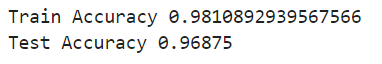Output using Dropout: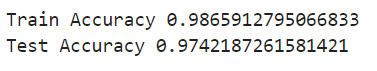We find that when we apply a dropout with probability p = 0.2, the test accuracy improves a bit and hence we can see a small regularizing effect here.

Conclusion:

There is an important observation to look at. Adding more data is not included in the techniques to solve underfitting. Indeed, if your data is lacking the decisive features to allow your model to detect patterns, you can multiply your training set size by 2, 5 or even 10, it won’t make your algorithm better! Unfortunately, it has become a reflex in the industry. No matter what the problem their model is facing, a lot of engineers think that throwing more data at it will solve the problem. When you know how time-consuming and expensive it can be to collect data, this is a mistake that can lead you in the wrong direction in your project. To solve overfitting, one must be well versed in playing with the neural network architecture in a clever way. Hence, being able to diagnose and tackle underfitting/overfitting is an essential part of the process of developing a good model.